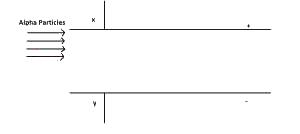# A stream of alpha particles is projected into an electric field between two plates X and Y as shown in the diagram above. Which of the following is correct?...

Question 1

A stream of alpha particles is projected into an electric field between two plates X and Y as shown in the diagram above. Which of the following is correct? The particles are